Industry Article

# Resolving the Signal Part 5: Understanding Effective Noise Bandwidth in Precision Delta-Sigma ADCs

## Part 5 of this 12-part series continues to explore effective noise bandwidth as it relates to delta-sigma ADCs and system-level design by stepping through a simple example using a two-stage filter to understand how to calculate the ENBW and how system changes affect the ENBW.

This 12-part article series focuses on the impact of noise in delta-sigma ADCs. Part 5 continues to explore effective noise bandwidth as it relates to delta-sigma ADCs and system-level design.

In part 4 of the Resolving the Signal series, I covered fundamental effective noise bandwidth (ENBW) topics including what ENBW is, why you need it, and where it comes from.

In part 5, I’ll continue to explore the ENBW as it relates to delta-sigma analog-to-digital converters (ADCs) and system-level design by stepping through a simple example using a two-stage filter to understand to important aspects of ENBW:

1. How to calculate the ENBW.
2. How system changes affect the ENBW.

### How to Calculate the ENBW

For this example, let’s use a simple, two-stage data-acquisition system comprising an anti-aliasing filter followed by a delta-sigma ADC with an integrated sinc filter, shown in Figure 1. As previously discussed, I’ll focus on these two filter types because they typically have the greatest impact on the overall ENBW, but this analysis is generally applicable to any type or number of additional filters.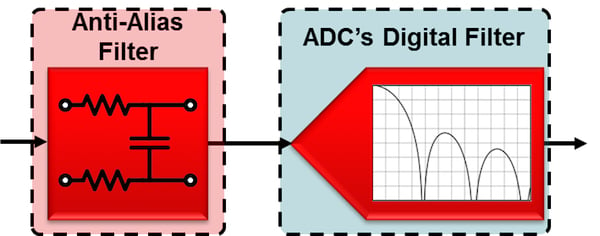##### Figure 1. Simplified data-acquisition system block diagram

For the anti-aliasing filter, let’s use a single-pole resistor-capacitor (RC) filter, since I already discussed how to calculate its ENBW in part 4 of this article series (reiterated below in Equation 1). Moreover, a delta-sigma ADC typically only requires a simple RC filter to provide sufficient anti-aliasing. Table 1 summarizes the chosen resistor and capacitor values for this example, though other valid combinations exist.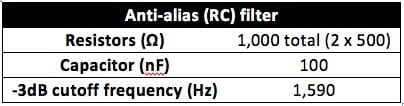##### Table 1. Anti-aliasing filter component values

With the passive component values selected, calculate the anti-aliasing filter’s ENBW using Equation 1: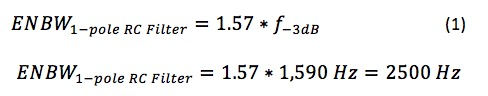Finally, plot the anti-aliasing filter’s response as shown in Figure 2, with the filter’s ENBW represented by the red shaded area.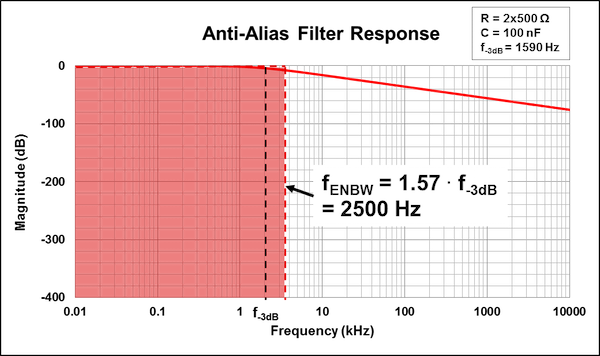##### Figure 2. Anti-aliasing filter response with ENBW highlighted

With a firm understanding of the anti-aliasing filter’s frequency response, the next step is to determine the response of the ADC’s sinc filter. For this example, let’s choose the 32-bit, low-noise ADS1262 from Texas Instruments (TI), although this analysis is generally applicable to any delta-sigma ADC. In this case, I’ll use the ADS1262’s sinc4 filter at a data rate of 60 samples per second (SPS). Figure 3 recreates a data-sheet plot for this filter’s frequency response at these settings (derived from the ADS1262 configuration tool).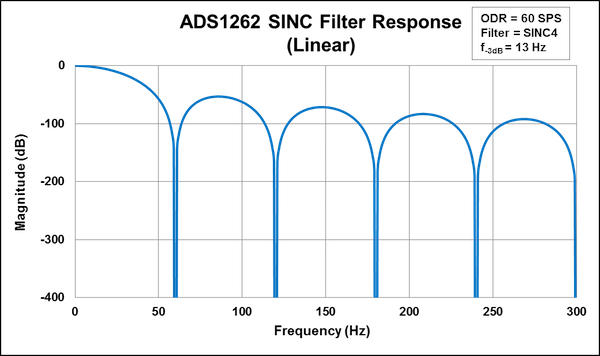##### Figure 3. ADS1262 SINC filter response — linear frequency scale, fmax=300Hz

One subtle but important difference between these two filter response plots is that the sinc filter plot (Figure 3) uses a linear frequency axis, while the anti-aliasing filter plot (Figure 2) has a logarithmic frequency axis. This disparity results from the slow data rates of most delta-sigma ADCs, where showing multiple frequency decades is generally unnecessary. Unfortunately, this choice complicates the process of combining the filters into the same plot.

Additionally, recall that a sinc filter response repeats indefinitely – it does not simply “stop” at 300Hz as it might appear in Figure 3.

If you take both of these issues into consideration and plot the sinc filter response on a logarithmic axis over a much wider frequency range, the result in Figure 4 is very different from the typical plot in most ADC datasheets.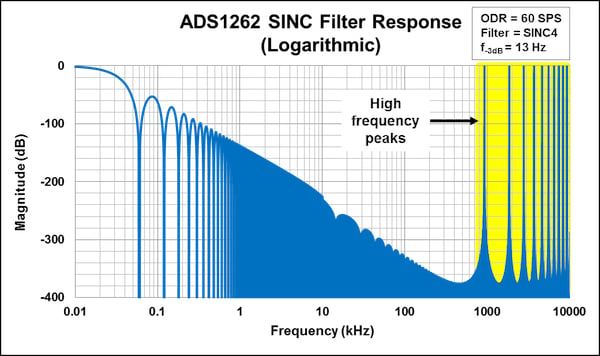##### Figure 4. ADS1262 SINC filter response — logarithmic frequency scale, fmax=10MHz

With a logarithmic axis plotted out to 10MHz, you can now see the high-frequency peaks indicating the filter response repeating. Why is this important? As a result of this repetition, integrating under the sinc filter’s frequency response curve yields an infinite ENBW (from a mathematical perspective, the sinc filter’s integral diverges at infinity). Figure 5 depicts a plot of both the anti-aliasing and sinc filter frequency responses and the ENBWs of each.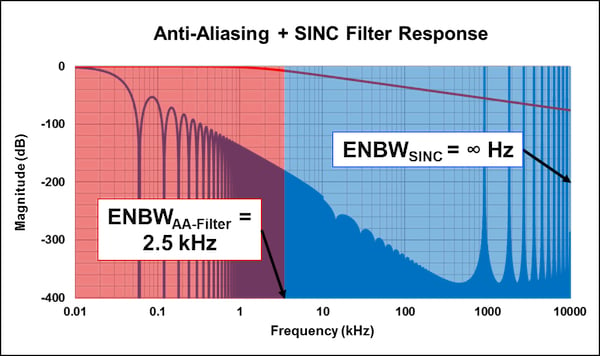##### Figure 5. ENBWs of SINC and anti-aliasing filters

How do you continue this analysis given the sinc filter’s infinite ENBW? You simply need to set limits on the integration. Generally, some multiple (1 or 2) of the modulator clock frequency (fMOD) is acceptable, but in this case you can use the anti-aliasing filter as the limit.

Now that both filters are magnitude plots (in decibels (dB) ) with the same x-axis scale, you can simply add them together to determine the overall system ENBW. This yields the filter response shown in Figure 6. Integrating the combined RC and digital filter response now results in an ENBW of 14Hz, orders of magnitude smaller than either filter by itself.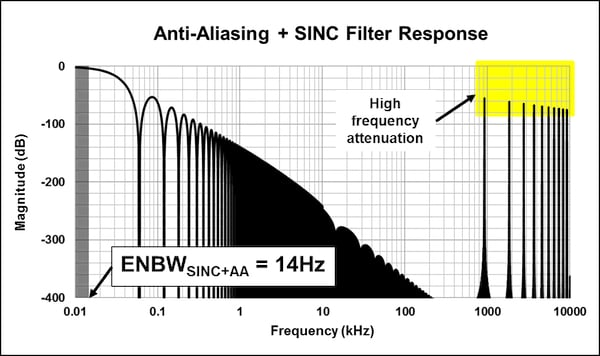##### Figure 6. System response of SINC and anti-aliasing filters

The narrower ENBW results from the anti-aliasing filter’s attenuation of the sinc filter noise power at higher frequencies, allowing less noise into the system. This also further explains why you don’t necessarily need to account for the infinite frequency response of the sinc filter. The anti-aliasing filter already removes much of the noise power associated with the high-frequency peaks occurring at multiples of fMOD that would otherwise fold back into the passband. Many analog designers assume that the anti-aliasing filter’s intended purpose is to remove low-frequency noise, which is generally the responsibility of the delta-sigma ADC’s digital filter.

If you followed this assumption and attempted to design an anti-aliasing filter with a very low cutoff frequency, you would generally need to use large resistor and capacitor values. However, large passive component values lead to longer signal settling time, which you generally want to avoid. Even if you could accept an additional settling time, ADC input leakage currents can cause significant offset voltages across large filter resistors, leading to system-level inaccuracy. Thus, it is actually to the system’s benefit that you only need to design anti-aliasing filters for high-frequency noise, as smaller passive components can help avoid the aforementioned issues.

### How System Changes Affect the ENBW

Suppose you now want to change the ADC’s sample rate or the anti-aliasing filter’s cutoff frequency. How does this affect the system ENBW? Intuitively, it would make sense that the filter with the smaller cutoff would dominate the ENBW calculation – as I have already shown – and this is in general correct. To illustrate this point, Table 2 lists all available digital filter output data rates of the ADS1262 as well as the corresponding system ENBW for a range of anti-aliasing cutoff frequencies. Table 2 also provides the ADC’s 3dB point, which effectively acts as its cutoff frequency.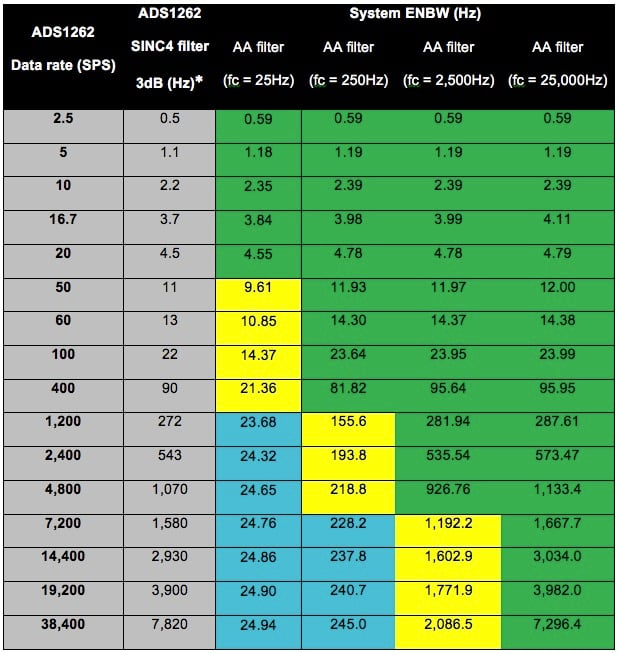##### Table 2. Effect of ADC data rate and AA-filter cutoff frequency on system ENBW.

Note: Results derived from the ADS1262 calculator tool.

The highlighted sections in Table 2 represent the following situations:

Green = The system's ENBW is within ~10% of the ADC's 3dB point.

Blue = The system's ENBW is within ~10% of the anti-aliasing filter's cutoff frequency.

Yellow = The system's ENBW is not within ~10% of the ADC's 3dB point of the anti-aliasing filter's cutoff frequency.

These relationships between the system ENBW and the individual filter cutoffs allow you to approximate the system ENBW instead of performing complex integrations, assuming that one of the conditions expressed in Equations 2 and 3 is true: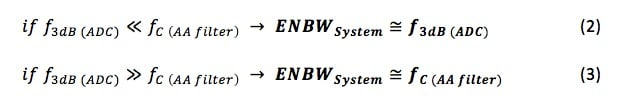If neither condition is true, such that f3dB(ADC) is relatively close to fc(AA filter), then it becomes necessary to perform the integrations outlined throughout this section. Moreover, these conditions would also generally apply to any number of additional filter stages as long as their cutoff frequencies were much larger than that of the ADC or anti-aliasing filter. In such cases, you wouldn’t have to calculate their ENBWs, making the analysis less complex.

In the next article of the “Resolving the Signal” series, I’ll continue the discussion of noise in delta-sigma ADCs by adding both integrated and external amplifiers to the signal chain.

### Key Takeaways

Here is a summary of important points to help better understand the ENBW in delta-sigma ADCs:

• The ENBW is typically dominated by the filter with the smallest cutoff frequency, which is typically an anti-aliasing filter or digital filter, especially for precision delta-sigma ADCs.
• Anti-aliasing filters exist to remove noise at high frequencies, not low frequencies.
• You can calculate the ENBW using direct integration or approximate it in most cases using the ADC’s 3dB point or the anti-aliasing filter’s cutoff frequency.

Industry Articles are a form of content that allows industry partners to share useful news, messages, and technology with All About Circuits readers in a way editorial content is not well suited to. All Industry Articles are subject to strict editorial guidelines with the intention of offering readers useful news, technical expertise, or stories. The viewpoints and opinions expressed in Industry Articles are those of the partner and not necessarily those of All About Circuits or its writers.